T
TeraPlot

# TeraPlot Graphing Software

TeraPlot scientific plotting software gives you everything you need for publication quality graph plotting in science and engineering. At its core is the ability to create 2D and 3D plots based on tabular data and/or mathematical expressions. Single plot types can be used individually within a graph, or multiple plots can be combined to construct graphs ranging from basic line plots, through 3D scatter plots, to complex 3D visualizations comprising analytical expressions, tabular data, 3D objects, annotation, and image overlay. In addition to these primary features, a range of capabilities such as flexible data import, and the ability to create graphs with just a few mouse clicks via graph wizards, make TeraPlot a highly effective scientific visualization tool. The program also provides curve fitting and data analysis features. Follow the link to the overview page for a more detailed outline..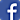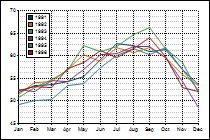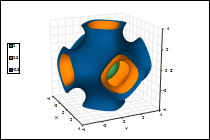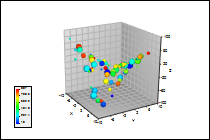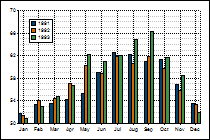## Evaluate TeraPlot

TeraPlot is a Microsoft Windows desktop application (Windows XP, Windows Vista, Windows 7, 8 and 10 ). Download a fully functional 30-day evaluation version..

## Surface Plotting

Plot and combine surfaces based on mathematical expressions, regular gridded data, or arbitrary (x, y, z) points. Surfaces can be plotted in cartesian, polar, and cylindrical coordinate systems. Display features include: colour mapping, texture mapping, overlay of secondary data, transparency, 3D contour display (lines and/or filled contours), general 3D transformation, wire frame display. Surface plotting is a primary requirement of any 3D graphing software and TeraPlot caters for virtually any scenario.

## Contour Plotting

Create contour plots based on functions, regular, or irregular data. Display contours and/or colourmapped filled levels. Add annotation labels with a wide range of label configuration options such as font, orientation, foreground and background colour. Because contour plots can be based on mathematical expressions, they can be used to draw implicit functions. For example, plotting xy - 5 = 0, would involve plotting z = xy - 5, and displaying the z = 0 contour value.

## Windows Store App

If you don't need the full power of a desktop application, and/or you'd like the flexibility of graphing software that runs on both a PC and a tablet, try TeraPlot LT, a Windows Store graph plotting app. TeraPlot LT allows you to create 2D line plots and 3D surface plots in various coordinate systems. As with the desktop version, TeraPlot LT plots can be based on mathematical expressions or supplied data. TeraPlot LT uses the muParser scripting engine.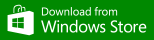## Scatter Plots

Teraplot graphing software allows you to create 2D scatter plots or 3D scatter plots in a range of coordinate systems. Use scatter point colour and size to visualise up to two further variables in addition to z value. Distinguish between data sets using different symbol types (e.g. sphere, cube, cone). Combine scatter plots with planes and text to create multidimensional analysis diagrams. Scatter plots can also be created with a few mouse clicks using Graph Wizards.

## Isosurfaces

Isosurfaces are surfaces of constant w for functions of the form w = f(x, y, z), i.e. isosurfaces are the 3d equivalent of contour plots. The data from which the isosurfaces are generated can be defined as a formula (analytical plot), or loaded from a file. For an analytical plot, the data is assumed to lie on a regular rectangular 3D grid. For data loaded from a file, the data can either lie on a regular grid, or arbitrary individual cells can be specified. Isosurfaces can also be used to plot implicit functions.

## Line/Series Plots

Create line plots based on arbitrary x-y values, or on Excel-like series/category data (series/category data can also be used to produce clustered bar plots, stacked bar plots, and area plots via graph wizards). Enhance 2D plots using symbols, captions, legends, shading and colourmaps. Combine plots based on mathematical functions and tabular data in the same graph. Place text at specific x-y positions using text plots. Copy graphs to the clipboard or export to image files.

## Graph Wizards

Create fully annotated, publication quality graphs with just a few mouse clicks via Graph Wizards. With graph wizards, graph creation is broken down into a set of input stages: plot choice, data input, plot parameter settings, and annotation (e.g. captions, colourmaps, legends) settings. Wizards are available for Excel-like series/category data, financial data, and XYZ point data. A graph created via a graph wizard can be used as-is, or as a starting point for a more complex graph.

## Function Plotting

TeraPlot brings the power of scripting to function graphing software. Using VBScript, function definitions can range from something as simple as the single line y = sin(x), to complex multi-line function definitions containing constants, variables and conditional expressions. Graphs of implicit functions can be easily created, and mathematical concepts explored with ease in a range of coordinate systems in both 2D and 3D.

## Flexible Data Import

Take advantage of a flexible range of data import features. Data can be entered into a plot manually, pasted from Excel, or imported into the graph from a previously created internal spreadsheet. These internal spreadsheets are created by importing data from character-delimited text files using an Excel-like data import feature. At each point in the plot creation, the spreadsheets are always available, providing easy access to your data.

## Data Analysis

Data analysis capabilities are provided in the form of statistical functions (Normal, Exponential, Lognormal, Weibull, Gamma, Binomial) and standard statistical analysis plots such as histograms (reporting Mean, Median, Variance, Standard Deviation, Skewness and Kurtosis), box plots, probability plots and curve fitting via linear regression. The program also features a general multidimensional nonlinear regression tool based on the Levenberg–Marquardt Method.

## Program Automation

Automation provides the ability to remotely start TeraPlot via a driver program written in e.g. VB.Net or C#. Much of the menu-driven functionality which would normally be accessed manually can then be accessed and manipulated programmatically. Controlling the program from a programming language provides a wide range of possibilities, from writing code which interactively controls the program via your own dialogs, to creating movies utilising any combination of program features you wish.

## Wide Range of Plots

Over 30 plot types, applicable over a wide range of disciplines, are available to meet your graphing software requirements. For 2D plotting there are basic line plots, series based plots such as stacked/clustered bars, statistical plots such as histograms and box plots, and contour plots. 3D plot types include surface plots based on regular or irregular data, scatter plots, 3D lines, bars, and object plots, which allow primitive objects to be laid out in 3D.

TeraPlot Graphing Software